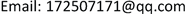OEOptoelectronics2164-5450Scientific Research Publishing10.12677/OE.2018.84024OE-27883OE20180400000_40407666.pdf工程技术 纠错码在无线激光通信中的应用 The Application of Error-Correcting Code in the Wireless Laser Communication 恒阔1*12金力12亚峰12龙超12中国电子科技集团公司第二十七研究所，河南 郑州null151120180804184191© Copyright 2014 by authors and Scientific Research Publishing Inc. 2014This work is licensed under the Creative Commons Attribution International License (CC BY). http://creativecommons.org/licenses/by/4.0/Copyright © 2018 by authors and Hans Publishers Inc.1. 引言

2. 大气湍流对激光通信的影响 [<xref ref-type="bibr" rid="hanspub.27883-ref1">1</xref>]

σ β 2 = 1.093 ⋅ L ⋅ C n 2 D 1 / 3

R max _ s g = R max _ s s 1 + σ β 2 R max _ s s 2

3. 纠错码技术 [<xref ref-type="bibr" rid="hanspub.27883-ref2">2</xref>] - [<xref ref-type="bibr" rid="hanspub.27883-ref9">9</xref>]

3.1. RS码

RS码首先由Reed和Solomon于1960年构造出来，是目前最为有效、应用最为广泛的差错控制编码之一，是一类具有很强纠错能力的多进制BCH码，它不仅可以纠正突发错误，还可以纠正随机错误。

RS码的一个重要性质是：真正的最小距离总是相同的。每种RS码都是一个最大距离的可分码，最小距离等于n − k − 1。当省掉RS码的某些信息符号后，分组长度缩短，但是最小距离并不减小，故任何一种缩短的码仍是一个最大距离可分码。

RS码的另一个重要形式是在其码字内的任何k个位置都是可用信息集合。(n, k)RS码中，输入信息分成km比特一组，每组包括k个符号，每个符号由m比特组成。RS码的编译码是基于一组码元而不是单独的0或1，这也是RS码纠错能力特别强的原因，这也使得RS特别适合处理突发错误。

3.1.1. RS编码原理

RS(n, k)码具有以下性质：

n为码长，k为信息位长，n − k为校验位长。一般 n = q − 1 ， n − k = 2 t ，q为有限域的阶数，是素数或素数的幂次，实用中一般取q为2的幂次。最小距离为 d min = 2 t + 1 ，t为设计的纠错能力。RS码主要是围绕生成多项式构成的，其生成多项式为：

g ( x ) = ( x − α m ) ( x − α m + 1 ) ⋯ ( x − α 2 t + m − 1 ) = a n − k x n − k + a n − k − 1 x n − k − 1 + ⋯ + a x x + a 0

a为GF(q)域上本原多项式的根，a的各次幂可构成扩域GF(qm)上的所有元素。

3.1.2. RS码译码原理

RS译码分为以下几步：

1) 伴随式的求解

g ( x ) = ( x − a 0 ) ( x − a 1 ) ⋯ ( x − a 2 t − 1 )

H = [ ( a 0 ) n − 1 ( a 0 ) n − 2 ⋯ ( a 0 ) 1 ( a 0 ) 0 ( a 1 ) n − 1 ( a 2 ) n − 2 ⋯ ( a 2 ) 1 ( a 2 ) 0 ⋮ ⋮ ⋱ ⋮ ⋮ ( a 2 t − 1 ) n − 1 ( a 2 t − 1 ) n − 2 ⋯ ( a 2 t − 1 ) 1 ( a 2 t − 1 ) 0 ]

S j = ∑ i = 1 t E l i x i j − 1 ,     j = 1 , 2 , ⋯ , 2 t

2) 差错位置多项式的确定

σ ( x ) = ( 1 − x 1 x ) ( 1 − x 2 x ) ⋯ ( 1 − x t x ) = 1 + σ 1 x + σ 2 x 2 + ⋯ + σ t x t

x 1 = ( x 1 σ ) − 1 , x 2 = ( x 2 σ ) − 1 , ⋯ , x t = ( x t σ ) − 1

3) 关键方程的求解

ME迭代算法可以简单描述为以下几个公式和约束条件的迭代。首先初始化： R 0 ( x ) = x 2 t ； Q 0 ( x ) = S ( x ) ； L 0 ( x ) = 0 ； U 0 ( x ) = 1 ；迭代后如果 deg ( R i ( x ) ) 或 deg ( Q i ( x ) ) 小于8，则算法迭代结束。此时 ω ( x ) = R i ( x ) ， σ ( x ) = L i ( x ) 。

4) 错误位置的确定

R ( x ) = r n − 1 x n − 1 + r n − 2 x n − 2 + ⋯ + r 1 x + r 0

σ ( α − ( n − 1 ) ) = σ ( α ) = 1 + σ 1 α + σ 2 α 2 + ⋯ + σ t α t = 0

5) 差错幅值的求解

s t = ∑ k = 1 7 Y k x k i

− σ ′ ( x i − 1 ) x i − 1 = ∏ j = 1 j ≠ i γ ( 1 − x j x − 1 ) ，同时得到

Y i x i x i − 1 ( − σ ′ ( x i − 1 ) ) ( x i − 1 ) = ω ( x i − 1 )

3.2. Turbo码

Turbo码是C. Berrou等人提出了一种新型的纠错码。它采用一种并行级联的方法实现了长码的编码，同时构造了相应的解码器来完成长码的解码，是一种实用的纠错码，它的性能非常逼近香农的性能界，使其在较低的信噪比条件下仍能够保持良好的性能。目前Turbo码在CDMA的应用得到各国学者的重视，Turbo迭代的思想已经渗透到物理链路层先进的信号处理技术的各个领域，它域调制、均衡、空时编码和OFDM相结合极大的促进了技术的进步和发展。

3.3. LDPC(低密度奇偶校验)码

LDPC码是Gallager于1962年提出的一种性能接近于香农限的好码，限于当时计算机水平和硬件技术LDPC码在很长一段时间内一直未受到人们重视，直到Berrou等人提出Turbo码后，LDPC码才重新引起人们的研究兴趣并成为研究的热点。计算机仿真结果表明，最好的非规则LDPC码(长度为106)可获得在BER = 10−6时仅偏离容量0.13 dB的性能，优于迄今所知道的最佳Turbo码，当码长为107码率为1/2时，其性能距香农限只差0.04 dB。

LDPC码的特点是：性能优于Turbo码，具有较大灵活性和较低的差错平底特性；译码复杂度低于Turbo码，且可实现完全的并行操作，在编码器和译码器之间不需要交织器，硬件复杂度低，因而适合硬件实现；吞吐量大，适合高码率的应用，极具高速译码潜力。此外，LDPC码在干扰、衰落等原因引起的突发误码情况下有更优良的性能。但LDPC码在硬件实现上难点是它的不规则结构，大部分的LDPC码的结构都是非常随机的，使得它的编码器要比Turbo码的复杂一些。另一个缺点是不能灵活地改变码率和码长，要改变通常需要重新构造奇偶校验矩阵和相应地生成矩阵，使得硬件改动困难。

4. RS码在无线激光通信链路中的应用

5. 结论